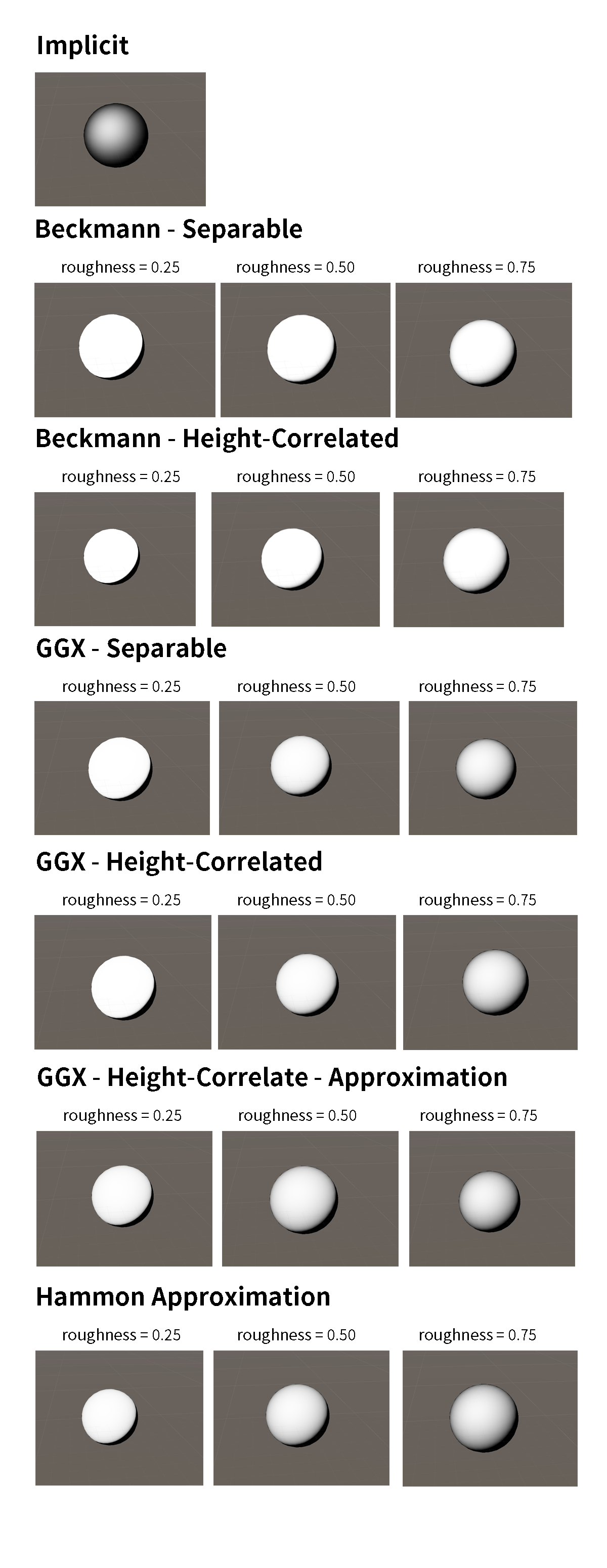• 《Real Time Rendering, 4th》- Chap. 9

## 基本性质

$\int_{\Omega} D(m) (v \cdot m) \mathrm{d} m = (v \cdot n)$

$\int_{\Omega} G_1(v, m) D(m) (v \cdot m)^+ \mathrm{d} m = (v \cdot n)$

## Implicit GSF

$G_2(l, v, m) = (l \cdot n) (v \cdot n)$

$V(l, v) = \frac{G_2(l, v, m)}{4(l \cdot n) (v \cdot n)}$

## Smith 遮蔽函数

$G_1(v, m) = \frac{\chi^+(v, m)}{1 + Ʌ(v)}$

$ɅɅ(v)$ 的定义可以看《Real Time Rendering, 4th》或毛神的白皮书（是的我没搞懂）。当法线分布是形状不变时，该函数有解析形式。对于我们在法线分布项中提过的两个形状不变的分布，其公式为：

Beckmann 分布：

$Ʌ(v) = \frac{\mathrm{erf}(a) - 1}{2} + \frac{1}{2a \sqrt{\pi}} \exp(-a^2) \\ a = \frac{1}{\alpha \tan \angle(v, n)} \\ \mathrm{erf}(x) = \frac{1}{\sqrt{\pi}} \int_{-x}^x \exp(-t^2) \mathrm{d}t$

$\mathrm{erf}(x)$ 是高斯误差函数，难以计算。其一个近似是：

$Ʌ(v) = \begin{cases} \frac{1 - 1.259a + 0.396a^2}{3.535a + 2.181a^2} & a < 1.6 \\ 0 & \mathrm{otherwise} \end{cases}$

GGX/TR 分布：

$Ʌ(v) = \frac{-1 + \sqrt{1 + 1 / a^2}}{2}$

## Smith 联合遮蔽阴影函数

Smith 联合遮蔽阴影函数（Smith Joint Masking-Shadowing Function）即同时考虑了出入射光的 $G_2$。其有四种形式：

$G_2(l, v, m) = G_1(l, m) G_1(v, m) = \frac{\chi^+(v \cdot m)}{1 + Ʌ(v)}\frac{\chi^+(l \cdot m)}{1 + Ʌ(l)}$

$G_2(l, v, m) = \frac{\chi^+(v \cdot m)\chi^+(l \cdot m)}{1 + Ʌ(v) + Ʌ(l)}$

$G_2 = \lambda(\phi)G_1(v, m)G_1(l, m) + (1 - \lambda(\phi)) \min(G_1(v, m) G_1(l, m))$

$\lambda(\phi) = \frac{4.41 \phi}{4.41 \phi + 1}$

$G_2 = \frac{\chi^+(v \cdot m) \chi^+(l \cdot m)}{1 + \max(Ʌ(v), Ʌ(l)) + \lambda(\phi) \min(Ʌ(v), Ʌ(l))}$

## GGX 高度相关的遮蔽阴影

$G_1(v, m) = \frac{2 (n \cdot v)}{(n \cdot v) + \sqrt{\alpha^2 + (n \cdot v)^2 (1 - \alpha^2)}} \\ G_2(l, v, m) = \frac{2(n \cdot v) (n \cdot l)}{(n \cdot l) \sqrt{\alpha^2 + (n \cdot v)^2 (1 - \alpha^2)} + (n \cdot v) \sqrt{\alpha^2 + (n \cdot l)^2 (1 - \alpha^2)}}$

$V(l, v) = \frac{0.5}{(n \cdot l) \sqrt{\alpha^2 + (n \cdot v)^2 (1 - \alpha^2)} + (n \cdot v) \sqrt{\alpha^2 + (n \cdot l)^2 (1 - \alpha^2)}}$

Hammon 在 2017 提出以下高度相关的 GGX 近似公式：

$V(l, v) = \frac{0.5}{\mathrm{lerp}(2 (n \cdot l) (n \cdot v), (n \cdot l) + (n \cdot v), \alpha)}$

## 可视化结果------ 本文结束，感谢您的阅读 ------